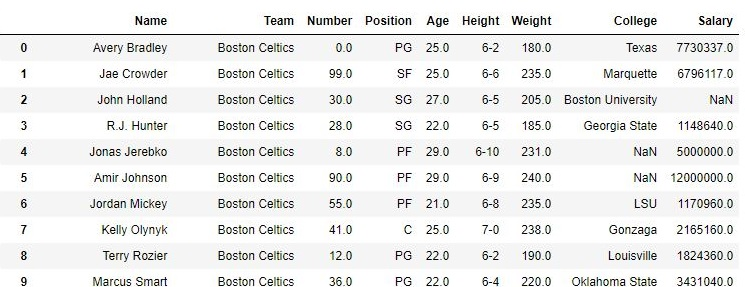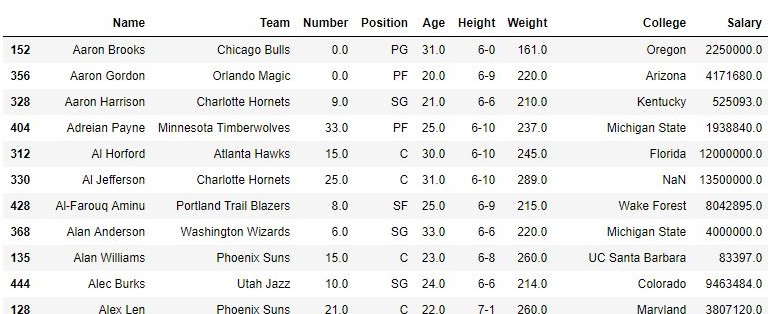# Python | Pandas Dataframe.sort_values() | Set-1

• Difficulty Level : Basic
• Last Updated : 20 Oct, 2021

Python is a great language for doing data analysis, primarily because of the fantastic ecosystem of data-centric Python packages. Pandas is one of those packages, and makes importing and analyzing data much easier.
Pandas sort_values() function sorts a data frame in Ascending or Descending order of passed Column. It’s different than the sorted Python function since it cannot sort a data frame and particular column cannot be selected.
Let’s discuss Dataframe.sort_values() Single Parameter Sorting:
Syntax:

DataFrame.sort_values(by, axis=0, ascending=True, inplace=False, kind=’quicksort’, na_position=’last’)

Every parameter has some default values except the ‘by’ parameter.
Parameters:

by: Single/List of column names to sort Data Frame by.
axis: 0 or ‘index’ for rows and 1 or ‘columns’ for Column.
ascending: Boolean value which sorts Data frame in ascending order if True.
inplace: Boolean value. Makes the changes in passed data frame itself if True.
kind: String which can have three inputs(‘quicksort’, ‘mergesort’ or ‘heapsort’) of algorithm used to sort data frame.
na_position: Takes two string input ‘last’ or ‘first’ to set position of Null values. Default is ‘last’.

Return Type:

Returns a sorted Data Frame with Same dimensions as of the function caller Data Frame.

For link to CSV file Used in Code, click here.
Example #1: Sorting by Name
In the following example, A data frame is made from the csv file and the data frame is sorted in ascending order of Names of Players.
Before Sorting-

## Python

 `# importing pandas package``import` `pandas as pd` `# making data frame from csv file``data ``=` `pd.read_csv(``"nba.csv"``)` `# display``data`

Output:After Sorting-

## Python

 `# importing pandas package``import` `pandas as pd` `# making data frame from csv file``data ``=` `pd.read_csv(``"nba.csv"``)` `# sorting data frame by name``data.sort_values(``"Name"``, axis ``=` `0``, ascending ``=` `True``,``                 ``inplace ``=` `True``, na_position ``=``'last'``)` `# display``data`

As shown in the image, index column is now jumbled since the data frame is sorted by Name.
Output:Example #2: Changing position of Null values
In the give data, there are many null values in different columns which are put in the last by default. In this example, the Data Frame is sorted with respect to Salary column and Null values are kept at the top.

## Python

 `# importing pandas package``import` `pandas as pd` `# making data frame from csv file``data ``=` `pd.read_csv(``"nba.csv"``)` `# sorting data frame by name``data.sort_values(``"Salary"``, axis ``=` `0``, ascending ``=` `True``,``                 ``inplace ``=` `True``, na_position ``=``'first'``)` `data``# display`

As shown in output image, The NaN values are at the top and after that comes the sorted value of Salary.
Output:My Personal Notes arrow_drop_up